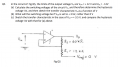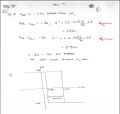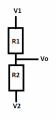# Schmitt trigger with different reference voltage

#### Mojo Pin__

Joined Apr 13, 2019
83
Hello, I am finding it difficult to understand how the switching voltage is calculated with a different reference voltage in part b of the question I posted. The professor has provided the solution but I don't understand the reasoning behind it.

For example, when calculating V+ and V-

V+ = ( Voutputhigh - Vref ) . R1/R2 + Vref

V- = ( Voutputlow - Vref ) . R1/R2 + Vref

What is happening in this equation?

Another question

Is R1/R2 equal to β?

I thought the gain of an ideal op amp was infinite, so why do we calculate β?

Any help would be greatly appreciated,

Thanks#### ericgibbs

Joined Jan 29, 2010
13,640
hi MP,
Look thru this PDF for hysteresis calculations, ask if you have a query, but post your results.
E

#### Attachments

• 178.6 KB Views: 14
•Mojo Pin__

#### Alec_t

Joined Sep 17, 2013
12,204
For example, when calculating V+ and V-
V+ = ( Voutputhigh - Vref ) . R1/R2 + Vref
V- = ( Voutputlow - Vref ) . R1/R2 + Vref
You are supposed to be calculating the input trigger thresholds under two conditions, namely when Vo is high (+12V) and when it is low (-12V). Is that what you mean by V+ and V-?
This question has nothing to do with calculating the op-amp gain, which here is assumed to be infinite.
R1 and R2 form a voltage divider which provides a fraction of the voltage difference (Vo-Vref) between its two ends.
The ratio R1/R2 is not correct for a voltage divider.

•Mojo Pin__

#### Mojo Pin__

Joined Apr 13, 2019
83
hi MP,
Look thru this PDF for hysteresis calculations, ask if you have a query, but post your results.
E

Thank you, I will have a go!

#### Mojo Pin__

Joined Apr 13, 2019
83
You are supposed to be calculating the input trigger thresholds under two conditions, namely when Vo is high (+12V) and when it is low (-12V). Is that what you mean by V+ and V-?
This question has nothing to do with calculating the op-amp gain, which here is assumed to be infinite.
R1 and R2 form a voltage divider which provides a fraction of the voltage difference (Vo-Vref) between its two ends.
The ratio R1/R2 is not correct for a voltage divider.

Ok thank you, I am finding this topic a bit confusing so thank you for clarifying about the gain.

V+ = ( Voutputhigh - Vref ) . (R1/(R1+R2)) + Vref
V- = ( Voutputlow - Vref ) . (R1/(R1+R2)) + Vref

Why do we subtract the reference voltage from the High and Low and then add it again, is this a voltage divider technique?

Thanks!

#### WBahn

Joined Mar 31, 2012
26,398
Ok thank you, I am finding this topic a bit confusing so thank you for clarifying about the gain.

V+ = ( Voutputhigh - Vref ) . (R1/(R1+R2)) + Vref
V- = ( Voutputlow - Vref ) . (R1/(R1+R2)) + Vref

Why do we subtract the reference voltage from the High and Low and then add it again, is this a voltage divider technique?

Thanks!
It is simply circuit analysis.

You have two resistors, R1 and R2, connected in series. You apply two voltages to the free ends, namely V1 to R1 and V2 to R2. What is the voltage, Vo, at the junction?•Mojo Pin__

#### Mojo Pin__

Joined Apr 13, 2019
83
It is simply circuit analysis.

You have two resistors, R1 and R2, connected in series. You apply two voltages to the free ends, namely V1 to R1 and V2 to R2. What is the voltage, Vo, at the junction?

View attachment 200034

Thank you for this response, it is becoming a bit clearer now,

So

V1 = 12V
V2 = 3.5V
R1 = 60kΩ
R2 = 12kΩ

so is it Vin = Vout ( R2 /( R2+R1) )

which is 12V = Vout ( 12k / 12k+60k)

but here is where I get stuck...

I'm sorry I don't understand how V2 is used properly in this equation, I know that the 3.5V must affect the value of Vin but why is it, for example, in the case of Voutputhigh:

Vout = ((12V-3.5V) . ( R2/(R2 + R1))) + 3.5V

I can't get my head around why V2 is added like this on to the equation

#### Alec_t

Joined Sep 17, 2013
12,204
You add on V2 because the potential divider between V1 and V2 is 'sitting on top of' V2, which may or may not happen to be 0V.
Think of the divider as being like a ladder. If the ladder is 4 metres long and its base is at sea level SL (our point of reference), when you (well, your feet actually) are half way up you are at a height of 2 metres above SL. If the base of the ladder were at 1000 metres above SL then you would be at a height of 1002 metres above SL. In other words, SL has been added to the height above the ladder base.

Last edited:
•Mojo Pin__

#### Mojo Pin__

Joined Apr 13, 2019
83
You add on V2 because the potential divider is 'sitting on top of' V2, which may or may not happen to be 0V.
You add on V2 because the potential divider is 'sitting on top of' V2, which may or may not happen to be 0V.

Thank you for that response, could you advise some reading or video material I could study so I can understand this concept a bit better please? most examples I find have the 2nd resistor connected to 0V.

In the Schmitt trigger case would an appropriate formula for this be:

Vout = ((V1 ± V2)(R2/(R2+R1))) +V2

And if this is appropriate would this be useful for most voltage divider circuits?

Thank you so much

#### Mojo Pin__

Joined Apr 13, 2019
83
You add on V2 because the potential divider between V1 and V2 is 'sitting on top of' V2, which may or may not happen to be 0V.
Think of the divider as being like a ladder. If the ladder is 4 metres long and its base is at sea level SL (our point of reference), when you (well, your feet actually) are half way up you are at a height of 2 metres above SL. If the base of the ladder were at 1000 metres above SL then you would be at a height of 1002 metres above SL. In other words, SL has been added to the height above the ladder base.
This just clicked, thank you. That's a great way of thinking about it

#### WBahn

Joined Mar 31, 2012
26,398
Thank you for this response, it is becoming a bit clearer now,

So

V1 = 12V
V2 = 3.5V
R1 = 60kΩ
R2 = 12kΩ

so is it Vin = Vout ( R2 /( R2+R1) )

which is 12V = Vout ( 12k / 12k+60k)

but here is where I get stuck...

I'm sorry I don't understand how V2 is used properly in this equation, I know that the 3.5V must affect the value of Vin but why is it, for example, in the case of Voutputhigh:

Vout = ((12V-3.5V) . ( R2/(R2 + R1))) + 3.5V

I can't get my head around why V2 is added like this on to the equation
The problem is that you are trying to force the use of a regurgitated equation in a situation in which it does not apply. This is due to relying on a bunch of memorized equations instead of understanding where those equations come from and what conditions or limitations existed.

Just apply the basic circuit analysis principles to the circuit. Let's get real explicit and down to basics.Do KVL around the circuit to find Io

V1 - Io·R1 - Io·R2 - V2 = 0

Io = (V1-V2)/(R1+R2)

Now what you are looking for is Vo (which is the same as Vb) in terms of Va and Vb (plus the circuit component values).

By the definition of the potential difference between two nodes, using double-subscript notation:

Vab = Va - Vb = Io·R1

This gives

Vb = Va - Io·R1

We could also use

Vbc = Vb - Vc = Io·R2

giving

Vb = Vc + Io·R2

Use either of these combined with Va = V1 and Vc = V2 and you get

Vo = V2 + (V1-V2)·R2/(R1+R2)

So how does this relate to the ubiquitous and of often-misapplied voltage divider formula?

The voltage divider equation is specific to the situation in which V2 = 0. Apply that constraint and, sure enough, you get the familier

Vo = V1·[R2/(R1+R2)]

Last edited:
•Mojo Pin__

#### Mojo Pin__

Joined Apr 13, 2019
83
The problem is that you are trying to force the use of a regurgitated equation in a situation in which it does not apply. This is due to relying on a bunch of memorized equations instead of understanding where those equations come from and what conditions or limitations existed.

Just apply the basic circuit analysis principles to the circuit. Let's get real explicit and down to basics.

View attachment 200052

Do KVL around the circuit to find Io

V1 - Io·R1 - Io·R2 - V2 = 0

Io = (V1-V2)/(R1+R2)

Now what you are looking for is Vo (which is the same as Vb) in terms of Va and Vb (plus the circuit component values).

By the definition of the potential difference between two nodes, using double-subscript notation:

Vab = Va - Vb = Io·R1

This gives

Vb = Va - Io·R1

We could also use

Vbc = Vb - Vc = Io·R2

giving

Vb = Vc + Io·R2

Use either of these combined with Va = V1 and Vc = V2 and you get

Vo = V2 + (V1-V2)·R2/(R1+R2)

So how does this relate to the ubiquitous and of often-misapplied voltage divider formula?

The voltage divider equation is specific to the situation in which V2 = 0. Apply that constraint and, sure enough, you get the familier

Vo = V1·[R1/(R1+R2)]
This is excellent, thank you so much. It is all becoming clearer now, I can't just plug values in to a formula without understanding the circuit. I'll try to keep this in mind when I work through the rest of these problems.

I never thought to redraw the circuit like that, it makes so much sense.

Thank you